Sep 13, 2022

# Mathematical DeFi Analysis of Uniswap - part 1

This section aims to provide a detailed, although partial, presentation of some salient features of Uniswap. The goal is to perform a surgical mathematical analysis of the protocol that allows to build sophisticated arbitrages on the top of it. Readers familiar with these subtle mechanisms can skip this section.

## Uniswap-(v1,v2)

### The names of the game

Uniswap is an autonomous decentralized market place as a collection of liquidity pools. A liquidity pool $\mathcal{L}^{ij} = \mathcal{L}(S_i \rightleftarrows S_j)$ is a smart contract representing a local market place that exchanges two tokens $S_i$ and $S_j$. The pool state $\mathcal{L}^{ij}{t} = \left( R{i}, , R_{j}, \kappa \right)t$ at aliquidity event time $t$ is defined by two reserves $R{i,t}, , R_{j,t} > 0$ of tokens held in the pool and a tension constant $\kappa > 0$ which is sat at the core of this constant market-maker product $x \times y = \kappa$. This protocol aims to preserve the product of the two reserves in the pool by adjusting the quantities of reserve from which a price is subsequently derived. The following result explores the theoretical root of this hyperbolic bonding curve.

Theorem 1. Let $\mathcal{L}(x \rightleftharpoons y)$ be an abstract pool (contract) exchanging two tokens $S_x$ and $S_y$ by controlling the reserves $x$ and $y$. A constant market-maker protocol $x \cdot y = \kappa$ is the protocol that keeps the reserves of the pool’s two tokens equal in value at the current price $p=\frac{y}{x}$ offered by the smart contract.

Proof. Let’s assume that some quantity $dy$ of token $S_y$ is sold in the pool, so that the corresponding reserve $y$ increases, in exchange of some quantity $dx$ of token $S_x$ so that the corresponding reserve $x$ decreases (as $dx<0$, the marginal quantity $-dx$ is sent out by the contract):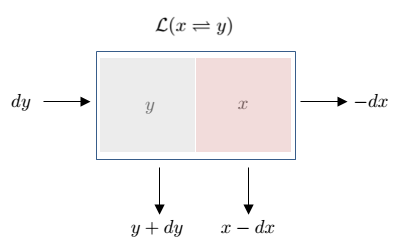Figure 1: Constant market-maker principle

Let $p=\frac{x}{y}$ the price of the asset-token $S_y$ in terms of the numeraire-token $S_x$ so that $x = p \cdot y$. Yet the marginal price offered by the contract is defined by $\lim_{dy\rightarrow 0} -\frac{dx}{dy}$. If the protocol controls for the fact that the pool’s two tokens are equal in value then it should maintain the relationship: $$\frac{x}{y} = -\frac{dx}{dy} \Leftrightarrow \frac{dy}{y} = - \frac{dx}{x}\Leftrightarrow\int{\frac{1}{y} dy} = -\int{\frac{1}{x} dx}$$ Solving both left-hand and right-hand sides gives $\ln y + c_y = - \ln x + c_x$ so that $\ln xy = c_x - c_y$ ad the results follows.

We can come back to a more practical analysis.

Definition 1. A pool $\mathcal{L}^{ij}$ exchanging two tokens $S_i$ and $S_j$ is at equilibrium at time $t$ if the internal pool state satisfies the condition: $$\label{pool_equilibrium_eq} R_{i,t} \cdot R_{j,t} = \kappa_t$$ where $\kappa_t$ is a pool’s constant at that time. Then, the relative equilibrium price of the asset-token $S_i$ expressed in terms of numeraire-token $S_j$ is: $$\label{price_constant_eq} p_{t} = \frac{R_{j,t}}{R_{i,t}}$$

This definition allows to link the pool state with the equilibrium price.

Lemma 2. When the pool state $\mathcal{L}^{ij}_{t}$ is at equilibrium, the reserves satisfy: $$\label{reserves_from_price_constant_eq} R_{i,t} = \sqrt{\frac{\kappa_t}{p_{t}}} \quad \text{and} \quad R_{j,t} = \sqrt{\kappa_t , p_{t}}$$

Traders interact with a liquidity pool $\mathcal{L}^{ij}$ by swapping $S_i \rightleftarrows S_j$. How does it work? Firstly, a trader observes the reserves in the pool and he is allowed to exchange some tokens $S_j$ he provides against some tokens $S_i$ he removes according to the state of the pool. Secondly, reserves are adjusted and the pool state state is updated, so the constant $\kappa$ is, and a new implied equilibrium is derived. Therefore, what traders ’see’ before swapping is described by the following graph: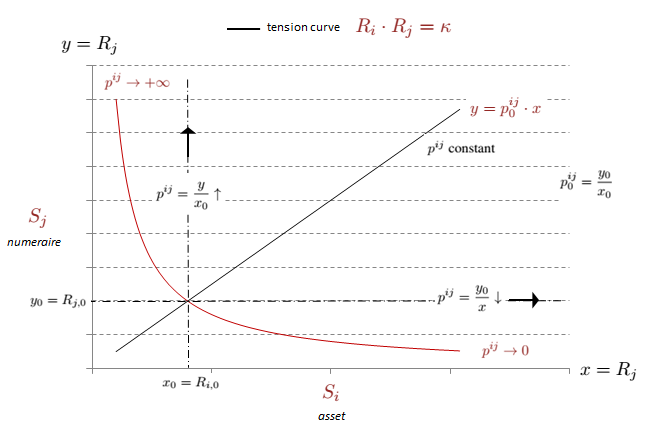Figure 2: Swapping $S_j = DAI$ against $S_i = ETH$

Definition 2. A swap $\Delta^{j \rightarrow i} = \Delta^{ij} = \left( +\Delta_i, , -\Delta_j \right)$ consists in exchanging a quantity $\Delta_j > 0$ (sell) of token $S_j$ against a quantity $\Delta_i > 0$ (buy) of token $S_i$ so that the reserves of the pool state become: $$\label{pool_state_evolution_constant_eq} \left( R_{i}, , R_{j}\right)t \longrightarrow \left( R{i} - \Delta_i, , R_{j} + \Delta_j \right){t+1}$$ This swap is valid if and only: $$\label{pool_state_constraint_constant_eq} \left( R{i,t} - \Delta_{i,t} \right) \cdot \left(R_{j,t} + (1-r)\Delta_{j,t} \right) = \kappa_t$$ where the transaction fee rate $r$ is essentially paid to the liquidity providers and solely taken from the quantity $\Delta_j > 0$ tokens $S_j$ which has been provided in exchange of $\Delta_i > 0$ tokens $S_i$. The two consecutive dates $t$ and $t+1$ at which the pool state changes are called liquidity events.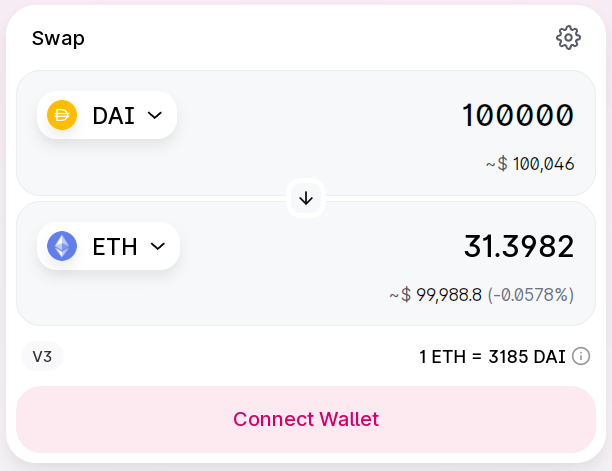Figure 3: Swapping $S_j$ against $S_i$

Corollary 3. When the transaction fees are not zero, so $r \not = 0$, the tension constant keeps being updated when the liquidity pool moves from a pool state $\mathcal{L}^{ij}{t}$ to another one $\mathcal{L}^{ij}{t+1}$ after every valid transaction:

$$\label{non_sontant_kappa_eq} \kappa_t = R_{i,t} \cdot R_{j,t} \mapsto \kappa_{t+1} = \left( R_{i,t} - \Delta_{i,t} \right) \cdot \left( R_{j,t} + \Delta_{j,t} \right)$$

As $S_j$ is swapped (sold) against $S_i$ (bought), it is natural to consider $S_j$ as a numeraire-token spent to acquire the asset-token $S_i$. We obtain a simple but important result:

Proposition 4. When a swap $\Delta^{ij} = \left( +\Delta_i, -\Delta_j\right)$ takes place at time $t$, the functional constraint imposed by a constant market-product implies a functional constraint between the ratio $\Delta_j / \Delta_i$ and the initial equilibrium price $R_{j,t} / R_{i,t}$: $$\label{functional_link_delta_reserve_eq} \forall t, ; \left {\begin{split} & \frac{\Delta_{i,t}}{R_{i,t}} = \frac{(1-r) \cdot \Delta_{j,t}}{R_{j,t} + (1-r) \cdot \Delta_{j,t}} \[5pt] & \frac{\Delta_{j,t}}{R_{j,t}} = \frac{\Delta_{i,t}}{(1-r) \cdot (R_{i,t}-\Delta_{i})} \end{split} \right.$$

Proof. Let $\mathcal{L}^{ij}{t}$ be a pool state at equilibrium, and let’s assume that a valid transaction $\Delta^{ij}{t}$ has just taken place at the liquidity event $t$. Following the definition ([pool_state_constraint_constant_eq]) and thanks to the definition (Definition 1) we deduce: $$\left( R_{i,t} - \Delta_{i,t} \right) \cdot \left(R_{j,t} + (1-r)\Delta_{j,t} \right) = R_{i,t} \cdot R_{j,t}$$ Solving this identity for $\Delta_{j,t}$ and $\Delta_{i,t}$ yields: \begin{aligned} \left { \begin{array}{lll} \Delta_{i,t} = R_{i,t} \cdot \frac{(1-r) , \Delta_{j,t}}{R_{j,t} + (1-r) , \Delta_{j,t}} \[5pt] \Delta_{j,t} = R_{j,t} \cdot \frac{\Delta_{i,t}}{(1-r)(R_{i,t} -\Delta_{i,t})} \end{array} \right.\end{aligned} and the result immediately follows.

It should be noticed that $\kappa$ is not always constant: when $r=0$, this AMM is said to be a constant product market because every swap $\Delta^{ij}$ changes the pool state so that: $$\left[ R_{i} \cdot R_{j} = \kappa \right]{t} \longrightarrow \left[\left(R_i - \Delta_i \right) \cdot \left( R{j} + \Delta_j \right) = \kappa \right]_{t+1} \Leftrightarrow \forall t, , \kappa_t=\kappa$$

Hence, the more the token $S_i$ is removed from the the liquidity pool, the higher the required quantity $\Delta_j$ is asked for the same quantity $\Delta_i$. Then the tension constant is automatically adjusted as the pool is evolving.

The protocol critically relies on the incentivization mechanism of the liquidity providers. Traders pay a trade fee rate $r=0.30%$. Yet, Uniswap v2 includes as well a protocol fee rate equal to 0.05% protocol fee that can be turned on: if it is turned on, a fee rate $r_{LP} = 0,25%$ is paid to liquidity providers, and the residual fee rate $r_{s} = 0.05%$ is sent to specific address in the factory contract. However, collecting this 0.05% fee would incur an additional gas cost.

So what? As a local market place, a liquidity pool $\mathcal{L}^{ij}$ hosts two types of participant.

• Traders: a trader swaps an amount $\Delta_j$ of token $S_j$ for and amount $\Delta_i$ of token $S_i$ or vice versa. When a trader exchanges $\Delta_j$ token $S_j$, the pre-trade reserves become: $$R_{j,t} \rightarrow R_{j,t} + (1-r)\Delta_j \quad \text{and} \quad R_{i,t} \rightarrow R_{i,t} - \Delta_i$$ where $\Delta_i$ is defined by the equation ([functional_link_delta_reserve_eq]). He pays $r\Delta_j$ transaction fee to liquidity providers in numeraire-token $S_j$. This fee is subsequently reinvested in the pool, so that the post-trade reserves turn out to be: $$R_{j,t+1} = R_{j,t} + \Delta_j \quad \text{and} \quad R_{i,t+1} = R_{i,t} - \Delta_i$$ The price $\breve{p}{t}(\Delta^{ij})$ at which the swap took place is: $$\breve{p}{t}(\Delta^{ij}) = \frac{\Delta_j}{\Delta_i} = \frac{\Delta_{j}}{R_{i,t}} + \frac{p_{t}}{(1-r)}$$

• Liquidity Provider: a liquidity provider adds or removes brings these two assets in order to enable the automatic market-making protocol. When he adds an amount $\Delta_j$ of token $S_j$ to reserve $R_{j,t}$, he needs to add also $\Delta_j \cdot R_i/R_j$ of token $S_i$ to reserve $R_i$. Then, the agent is rewarded in liquidity tokens $S_{\text{uni}}$: $$\Delta_{\text{uni}} = \frac{\Delta_j}{R_j} \cdot R_{\text{uni}}$$ where $R_{\text{uni}}$ denotes the total amount of liquidity tokens given by the pool’s contract. Conversely, when he removes liquidity from the pool, the amount $\Delta_{\text{uni}}$ is burnt and the pool’s contract gives the liquidity provider: $$\Delta_i = \frac{\Delta_{\text{uni}}}{R_{\text{uni}}} \cdot R_i \quad \text{and} \quad \Delta_j = \frac{\Delta_{\text{uni}}}{R_{\text{uni}}} \cdot R_{j,t}$$

When the swap takes place, the equilibrium price of the liquidity pool is given by the equation ([price_constant_eq]), so that: $$\lim_{\Delta_j \rightarrow 0} \frac{\breve{p}{t}(\Delta^{ij})}{p_t} = \frac{1}{1-r}$$ Hence, when $S_i$ is bought at time $t$, traders pay a fixed spread $p_t/(1-r)$, while when $S_i$ is sold, traders pay a fixed spread $(1-r)p_t$. It clearly appears that the price impact of a marginal increase of $\Delta_j$ is $\frac{\partial\breve{p}{t}(\Delta^{ij})}{\partial\Delta_j} = R_{i,t}$, which is decreasing in the size of the liquidity pool.

Example. Let’s consider a liquidity pool $\mathcal{L}^{ETH,DAI}$ with $R_{DAI,t}=10,000$ and $R_{ETH,t}=4$, so that the equilibrium price of the asset-token ETH in numeraire-token DAI is $p_t=2,500$. Let’s consider a trader who wants to swap $\Delta_{DAI} = 1,500$ DAI (sell) for ETH (buy); invoking the equation ([functional_link_delta_reserve_eq]), the amount of ETH he receives is $\Delta_{ETH} \simeq 0.5204$. This trader pays a transaction fee $r\Delta_{DAI} = 4.50$ DAI collected by liquidity providers. When this fee is re-invested in the pool, the post-trade reserves become $R_{DAI,t+1} = 11,500$ and $R_{ETH,t+1} \simeq 3.4796$. The average price paid during this swap is $\breve{p}_{t}(\Delta^{ij}) \simeq 2,882.5226$ DAI or 1 ETH.

### Impermanent loss

The concept of impermanent loss plays a central role in AMMs and has received multiple attentions. Yet, it should be more accurate to speak about a measure of relative performance as it refers to the difference between the value of the current fee adjusted position in the AMM protocol and the value stemming from a long position that was originally contributed. But, because the ’reality’ in a given AMM is always driven by an algorithmic principle by forcing a token pair to move along a smooth curve that aims to capture tension between supply and demand, it would be probably more accurate to speak about tension loss: a loss stemming from a line of tension between two tokens. We obtain the following definition:

Proposition 5. The impermanent or tension loss that happens in a liquidity pool $\mathcal{L}^{i,j}$ measures how the wealth process generated by a liquidity provider strategy deviates from the wealth process generated by a hodl strategy. The initial tension loss $\ell^{ij}{t,t+1}$ measures this relative performance with respect to the initial liquidity provider wealth, while the terminal tension loss $\breve{\ell}^{ij}{t,t+1}$ measures this relative performance with respect to the final hodl investor wealth. Without transaction fee, these impermanent losses are: $$\label{tension_loss_eq} \left { \begin{split} & \breve{\ell}{t,t+1}^{ij} = 2, \frac{\sqrt{\delta}}{\delta + 1} - 1 \[5pt] & \ell{t,t+1}^{ij} = \sqrt{\delta} - \frac{\delta+1}{2} - 1 \end{split} \right.$$

where $\delta = p_{t+1}/p_{t}$ is the percentage change of the price process of $S_j$ with respect to $S_i$.

Proof. Let $V_{t}$ be the wealth process of a liquidity provider strategy, and let $V^{}{t}$ be the one of a hodl strategy, both of them being expressed in the same numeraire $S_j$ (that correspond to the sold token in a transaction swap). Let’s consider a liquidity provider who has provided $q%$ of the total amount of tokens $S_i$ and $S_j$ held in the pool, so that $q R{i,t}$ and $q R_{j,t}$ are his respective holdings. Finally let’s consider a hodl investor with the same amount of tokens invested in another place. We assume that the pool is always arbitrage free after two event dates so that the ’market reality’ for both of them is given by the protocol’s oracle. Assuming that the pool state $(R_{i,t}, , R_{j,t})$ is at equilibrium at initial time $t$ (and $q=1$ without loss of generality) we dedeuce from the equation ([reserves_from_price_constant_eq]) $V_{t} = V^{}{t} = \left( R{j,t} + p_{t}R_{i,t} \right) = 2 \sqrt{\kappa , p_{t}}$, because $\forall t, , \kappa_t=\kappa$ without transaction fees. Now, let’s assume that a new transaction has just taken place in the pool at the price $p_{t+1}$; the liquidity provider has to adjust his holdings as prescribed by the new pool state $(R_{i,t+1}, , R_{j,t+1})$: $$V_{t+1} = R_{j,t+1} + p_{t+1}R_{i,t+1} = 2\sqrt{\kappa , p_{t+1}}$$ However, the hodl investor has nothing to do nothing and his wealth process becomes: $$V^{}{t+1} = R{j,t} + p_{t+1}R_{i,t} = \sqrt{\kappa p_{t}} + \sqrt{\frac{\kappa}{p_{t}}}p_{t+1}$$ The relative performance of these two wealth processes is simply: $$\begin{split} L_{t,t+1} &= \left( V_{t+1} - V^{}{t+1} \right) - \left( V{t} - V^{}{t} \right) \ &= 2\sqrt{\kappa , p{t+1}} - \sqrt{\kappa p_{t}} - \sqrt{ \frac{\kappa}{p_{t}}}p_{t+1} \end{split}$$ By definition, the tension loss is $\ell_{t,t+1}^{ij} = \frac{L_{t,t+1}}{V_{t}} = \sqrt{\frac{p_{t+1}}{p_{t}}} - \frac{1}{2} \left( 1 + \frac{p_{t+1}}{p_{t}} \right)$ and the terminal tension loss is: $$\breve{\ell}{t,t+1}^{ij} = \frac{L{t,t+1}}{V^{}{t+1}} = 2, \frac{\sqrt{\frac{p{t+1}}{p_{t}}}}{1+\frac{p_{t+1}}{p_{t}}} - 1$$ and the result immediately follows with $\delta=p_{t+1}/p_t$.

The simulation of these tensions losses reveals something critical: compared with a hodl strategy, a liquidity provider strategy looks like a with respect to the price process $p_{t}$ as it is illustrated below: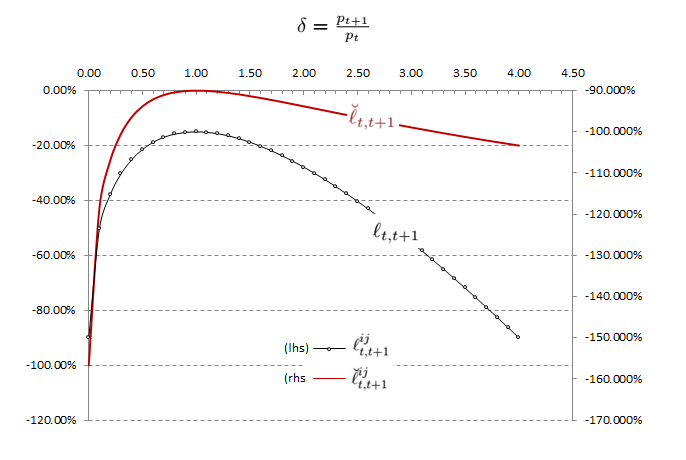Figure 4: Tension loss with respect to the price ratio $\delta$

Hence, the ’carry’ or ’yield’ extracted by providing liquidity in a pool can be perceived as an enhanced digital money market strategy which sells synthetic risk.

We can derive the Uniswap-v2 tension with transaction fee.

Theorem 6. The tension loss $\breve{\ell}^{ij}_{t,t+1}$ measured by the relative performance of a liquidity providing strategy in the liquidity pool $\mathcal{L}^{ij}$ with respect to the final hodl strategy whit transaction fee $r>0$ is: $$\label{uniswapv2_tension_loss__withfee_eq} \breve{\ell}_{t,t+1}^{ij}= \left { \begin{split} & \frac{(2-r)\sqrt{\delta}-r\delta}{(1-r)(1+\delta)}-1 & \quad \text{if} \quad \delta \leq 1 \[5pt] &\frac{(2-r)\sqrt{\delta}-r}{(1-r)(1+\delta)}-1 & \quad \text{if} \quad \delta > 1 \end{split} \right.$$

where $\delta=\delta_{t,t+1}=\frac{p_{t+1}}{p_{t}}$ is the percentage change of the price process of $S_j$ with respect to $S_i$ between these two dates.

Proof. The initial portfolio value of a hodl strategy is $V_{t}^{} = R_{i,t},p_t + R_{j,t}$, the two holdings remaining fixed. The pool $\mathcal{L}^{ij}$ being at equilibrium at time $t$, we know that $R_{i,t}=\sqrt{\kappa_t/p_t}$ and $R_{j,t}=\sqrt{\kappa_t p_t}$. If the ‘external price’ of the asset-token $S_i$ in numeraire-token $S_j$ (given by another oracle such as a centralized liquid exchange) suddenly changes to $p_{t+1}’ = \delta p_t$, then this value becomes: $$V_{t+1}^{} = \sqrt{\frac{\kappa_t}{p_t}} \delta p_t + \sqrt{\kappa_t p_t}=(1+\delta)\sqrt{\kappa_t p_t}$$ Similarly, the initial value of the liquidity providing portfolio is $V_t=R_{i,t}p_t + R_{j,t}$ so that $V_{t}^{*}=V_{t}$.

Let’s suppose that this external $p_{t+1}’$ increases, so $\delta>1$. It is important to notice that Uniswap sequentially updates its state variables: firstly $(R_{i,t},R_{j,t}) \rightarrow (R_{i,t+1},R_{j,t+1})$ and secondly $\kappa_{t} \rightarrow \kappa_{t+1}$ and internal price $p_t\rightarrow p_{t+1}$. The reserves have to be adjusted to reflect this new price so that $R_{i,t} \rightarrow R_{i,t} + \Delta R_i$ with $\Delta R_i<0$ and $R_{j,t} \rightarrow R_{j,t} + (1-r) \Delta R_j$ with $\Delta R_j>0$ and $R_{u,t+1} - R_{u,t} = \Delta R_u$ for $u=i,j$. We derive the two conditions: $$\left { \begin{split} &R_{i,t} + \Delta R_i = \sqrt{\frac{\kappa_t }{\delta p_t}} \[5pt] &(1-r)R_{j,t+1} + rR_{j,t} = \sqrt{\kappa_t \delta p_t} \end{split} \right. \Leftrightarrow \left { \begin{split} &R_{i,t+1} = \sqrt{\frac{1}{\delta}} \sqrt{\frac{\kappa_t }{ p_t}} \[5pt] &R_{j,t+1} = \frac{\sqrt{\kappa_t p_t}}{1-r} \left( \sqrt{\delta} - r \right) \end{split} \right.$$ We deduce: $$V_{t+1} = R_{i,t+1} \delta p_t + R_{j,t+1} = \frac{\sqrt{\kappa_t p_t}}{1-r} \left((2-r)\sqrt{\delta} - r \right)$$ so that $$\frac{V_{t+1}}{V_{t+1}^{*}} - 1 = \frac{(2-r)\sqrt{\delta} - r}{(1-r)(1+\delta)} - 1$$

Conversely, lets suppose that the price $p_{t+1}’$ decreases, so $\delta\leq1$. The adjustment of reserves to reflect this new price is $R_{i,t} \rightarrow R_{i,t} + (1-r)\Delta R_i$ and $R_{j,t} \rightarrow R_{j,t} + \Delta R_j$ with $\Delta R_i>0$ and $\Delta R_j<0$. We derive two conditions: $$\left { \begin{split} &(1-r)R_{i,t+1} + rR_{i,t} = \sqrt{\frac{\kappa_t}{\delta p_t}} \[5pt] &R_{j,t} + \Delta R_j = \sqrt{\kappa_t\delta p_t} \end{split} \right. \Leftrightarrow \left { \begin{split} &R_{i,t+1} = \frac{1}{1-r} \sqrt{\frac{\kappa_t}{p_t}} \left( \frac{1}{\sqrt{\delta}} - r \right) \[5pt] &R_{j,t+1} = \sqrt{\kappa_t p_t} \sqrt{\delta} \end{split} \right.$$

We deduce: $$V_{t+1} = R_{i,t+1} \delta p_t + R_{j,t+1} = \frac{1}{1-r} \sqrt{\kappa_tp_t} \left( (2-r)\sqrt{\delta} - r\delta\right)$$ so that $$\frac{V_{t+1}}{V_{t+1}^{*}} - 1 = \frac{(2-r)\sqrt{\delta} - r\delta}{(1-r)(1+\delta)} - 1$$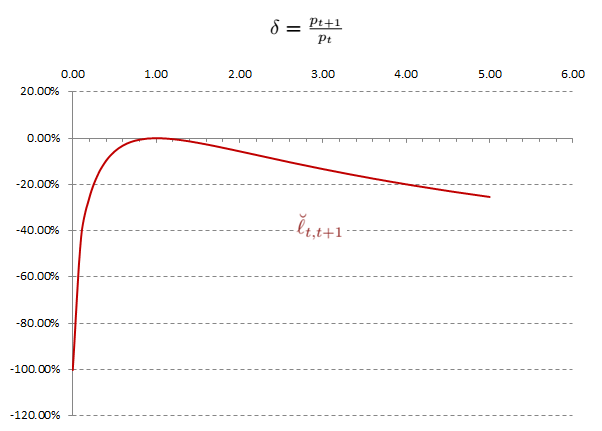Figure 5: Uniswap-v2 tension loss with transaction fees*

There exists a small region $1 \leq \delta \leq (1-r)^{-1}$ within which the impermanent loss is actually positive: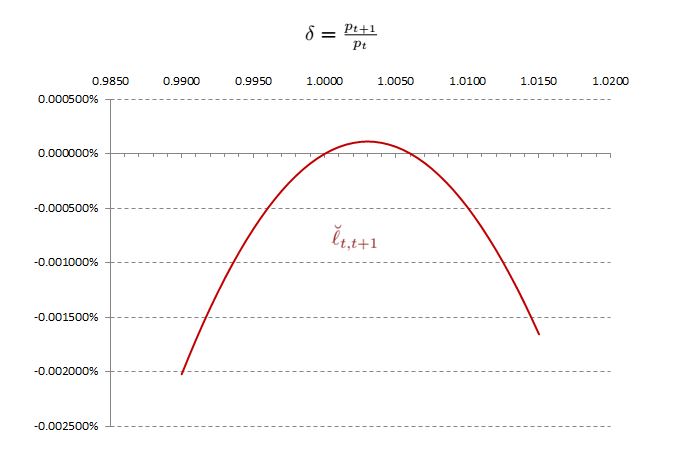Figure 6: Uniswap-v2 tension loss for $1 \leq \delta \leq (1-r)^{-1}$

### Optimal classical arbitrage

Uniswap can be seen as a decentralized market place $\mathbb{M}{\text{U}}$ embedded in an ecosystem of other centralized and decentralized markets places $\mathbb{M} = \mathbb{M}{\text{S}} \cup \mathbb{M}{\text{B}} \cup \cdots$ such as Sushiswap, Binance and so on and so forth. As such, all these market places are different oracles that broadcast token prices in $\mathbb{M}$ and can be subject to relative price discrepancies: the price $p{\mathbb{M}{\text{U}},t}$ of a token pair $(S_i,S_j)$ in $\mathbb{M}{\text{U}}$ can diverge from its price $p_{\mathbb{M}_{o},t}$observed in some other $\mathbb{M}$’s exchanges. Studying how to arbitrage these price discrepancies is useful to build a better understanding of the AMM’s dynamics.

The most classical DeFi arbitrage is exactly the similar to the one that used to prevail finance when a simultaneous purchase and sale of the same asset in different markets takes place in order to profit from small price differences.

Proposition 7. In a DeFi standard price arbitrage, an arbitrager seeks to maximise the profit that stems from the following sequence:

• Swapping $\Delta_{j} \rightleftharpoons \Delta_i$, of $S_j$-token against $S_i$-token in Uniswap market $\mathbb{M}_{\text{U}}$

• Exchanging $\Delta_i \rightleftharpoons \Delta_{j}^{’}$ in another market $\mathbb{M}{0}$ where the the $S_j$-asset price expressed in the $S_i$-numeraire token is $p{\mathbb{M}_o}$.

When $\Delta_{j}^{’} - \Delta_{j} > 0$ the profit is positive and there is an arbitrage opportunity between these two market places. The arbitrager has to solve the optimal arbitrage problem (without denoting the time-stamp subscript $t$):

$$\label{standard_arbitrage_problem_eq} \left { \begin{split} & \max_{\Delta_i, \Delta_j} \quad p_{\mathbb{M}_o} , \Delta_i - \Delta_j \[2pt] & \text{s.t.} \[2pt] & (R_i - \Delta_i)(R_j + (1-r)\Delta_j) = \kappa \quad \text{and} \quad \Delta_i, ; \Delta_j \geq 0 \end{split} \right.$$

whose the solution is:

$$\label{standard_arbitrage_solution_eq} \left { \begin{split} & \Delta_{i}^{} = \max \left( R_i - \sqrt{\frac{\kappa}{(1-r), p_{\mathbb{M}{o}}}}, , 0 \right) \[5pt] & \Delta{j}^{} = \max \left( \sqrt{\frac{\kappa , p_{\mathbb{M}_{o}}}{1-r}} - \frac{R_j}{1-r}, 0 \right) \end{split} \right.$$

Proof. Let’s assume that the arbitrager can exchange $\Delta_{j} \rightleftharpoons \Delta_i$ in $\mathbb{M}{\text{U}}$; as these two reserves satisfy the identity ([pool_equilibrium_eq]),we have: $$\Delta_j = \frac{1}{1-r} \left( \frac{\kappa}{R_i - \Delta_i} - R_j \right)$$ that makes clearly appear $\Delta_j$ as a convex function $\Delta_i$. Let $p{\mathbb{M}0}$ be the reference price of the token pair in a centralized exchange so that $\Delta{j}^{’} = p_{\mathbb{M}0} , \Delta_i$ represents the fair equivalent amount of tokens $S_j$ in $\mathbb{M}0$. The arbitrager has to solve the convex optimisation problem: \begin{aligned} \left { \begin{array}{lll} \max{\Delta_i} ; p{\mathbb{M}0} , \Delta_i - \frac{1}{1-r} \left( \frac{\kappa}{R_i - \Delta_i} - R_j \right) \[5pt] \text{s.t.} ; \Delta_i \geq 0 \end{array} \right.\end{aligned} The optimal solution of this unconstrained problem over the interval $[0, +\infty[$ is a zero of the derivative of the objective function and few lines of simple algebra yield: $$\Delta{i}^{} = \max \left( R_i - \sqrt{\frac{\kappa}{(1-r)p_{\mathbb{M}0}}}, , 0 \right)$$ The first constraint that ensures that the swap is a valid transaction in the liquidity pool provides the identity that about the optimal amount of $S_j$-token to trade: $$R{j}^{} + (1-r)\Delta_j = \frac{\kappa}{R_i - \Delta_{i}^{}}$$ so that: $$\Delta_{j}^{} = \max \left( \sqrt{\frac{\kappa , p_{\mathbb{M}_0}}{1-r}} - \frac{R_j}{1-r}, 0 \right)$$

We deduce the following simple corollary.

Corollary 8. In a standard DeFi arbitrage the optimal solution is $\left( \Delta_{i,t}^{}, \Delta_{j,t}^{*} \right) = (0,0)$ if and only if: $$\label{nonarbitrage_condition_eq} p_{\mathbb{M}{U},t} \geq (1-r) , p{\mathbb{M}_{o},t}$$

Proof. From the previous proposition, we know that the optimal solution satisfies: $$\Delta_{i,t}^{} = \max \left( R_{i,t} - \sqrt{\frac{\kappa}{(1-r), p_{\mathbb{M}{o}}},t}, , 0 \right)$$ so that: $$\Delta{i,t}^{} \geq 0 \Leftrightarrow R_{i,t} \geq \sqrt{\frac{\kappa}{(1-r), p_{\mathbb{M}{o},t}}}$$ But as the pool is at equilibrium we know that $R{i,t} , R_{j,t} = \kappa_t$, the results follows

Therefore, it is impossible to deploy such a standard arbitrage if the market is infinitely liquid. This notion of arbitrage is helpful to measure how the Uniswap oracle $\mathbb{M}{U}$ can deviate from another centralized exchange oracle $\mathbb{M}{o}$. The following result measures the infinitesimal variations that leave the liquidity pool at equilibrium.

Lemma 9. *The marginal equilibrium price of the $S_i$-asset in the $S_j$-numeraire is the price $\gamma^{ij}{t}=\gamma_t$ of an infinitesimal swap transaction that leaves the pool $\mathcal{L}^{ij}$ at equilibrium so that: $$\label{marginal_equilibrium_price_lem} \gamma_t = (1-r)^{-1} , p{t}$$

Proof. Let’s consider a valid swap transaction $S_j \rightleftharpoons S_i$, we know that the protocol modifies the tension constant so that $\kappa_t = (R_{i,t}-\Delta_{i,t})(R_{j,t}+(1-r)\Delta_{j,t})$. We look for an infinitesimal swap $\Delta_{i,t}$ change that leaves the tension constant unchanged, because $\Delta_{j,t} = h(\Delta_{i,t}) = \frac{1}{1-r} \left( \frac{\kappa}{R_{i,t} - \Delta_{i,t}} - R_{j,t}\right)$ we deduce: $$\dv{\Delta_{j,t}}{\Delta_{i,t}} = (1-r)^{-1} , \kappa_t , \left( R_{i,t} - \Delta_{i,t} \right)^{-2}$$ so that: $$\lim_{\varepsilon\rightarrow 0} \left(\dv{\Delta_{j,t}}{\Delta_{i,t}}\right){\Delta{i,t}=\varepsilon} = (1-r)^{-1} , \frac{\kappa_t}{R_{i,t}^{2}}$$ As $\kappa_t = R_{i,t} R_{j,t}$, the result follows.

Proposition 10. *Let $\mathcal{L}^{ij}$ be a liquidity pool in the Uniswap DeFi market $\mathbb{M}{U}$, and let $p{\mathbb{M}{U},t}$ the exchange price of the asset-token $S_i$ in the numeraire-token $S_j$ in the pool. Let $p{\mathbb{M}{o},t}$ the price of this token pair in another centralized exchange $\mathbb{M}{o}$. Then the deviations of $p_{\mathbb{M}{U},t}$ from $\mathbb{M}{o}$ are constrained by the level of trading fee rate $r$ which is paid to liquidity providers: $$\label{uniswap_price_deviation_prop} (1-r) , p_{\mathbb{M}{o},t} \leq p{\mathbb{M}{U},t} \leq (1-r)^{-1} , p{\mathbb{M}_{o},t}$$

Proof. In the previous proposition ([nonarbitrage_condition_eq]) it has been proved that the non-arbitrage hypothesis leads to $(1-r) , p_{\mathbb{M}{o},t} \leq p{\mathbb{M}_{U},t}$. Applying the very same construction by swapping $S_j$ against $S_i$ in the pool yields the desired result.

It should be noticed that if $r$ is small (in Uniswap-v3 the transaction fee rate for stable coins is $r=0.01%$) then a Taylor expansion gives the approximation: $$(1-r) , p_{\mathbb{M}{o},t} \leq p{\mathbb{M}{U},t} \lesssim (1+r) , p{\mathbb{M}_{o},t}$$

### Dynamical properties of the tension constant

As it has been proved above, the tension constant is not a ’constant’ of the protocol as it is kept being updated after every swap that takes place in the pool. We obtain the following formal result.

Proposition 11. *The tension constant of a liquidity pool $\mathcal{L}^{ij}$ is a non-decreasing process and is strictly increasing if $r>0$: $$\label{tension_constant_evolution_eq} \forall t, ; \kappa_{t+1} \geq \kappa_{t}$$

Proof. Let $\Delta^{ij}{t}$ be valid swap transaction that happens at the liquidity event $t$ swapping (sell) $\Delta{j,t}$ amount of $S_j$-tokens against (buy) $\Delta_{i,t}$ amount of $S_i$-tokens. The life cycle of the pool as a sequential discrete machine is the following:

• The swap is such that $\kappa_{t} = (R_{i,t} - \Delta_{i,t})\cdot(R_{j,t} + (1-r)\Delta_{j,t})$.

• At $t+1$, the reserves are updated, $R_{i,t+1} = R_{i,t} - \Delta_i$ and $R_{j,t+1} = R_{j,t} + \Delta_j$, and a new constant $\kappa_{t+1} = R_{i,t+1} \cdot R_{j,t+1}$ is derived accordingly.

Because $0 \leq 1-r \leq 1$, we obtain $\kappa_{t+1} \geq (R_{i,t} - \Delta_{i,t})\cdot(R_{j,t} + (1-r)\Delta_{j,t})$ and the result follows.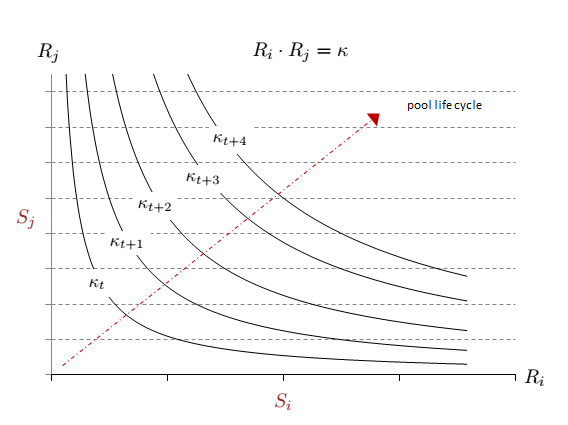Figure 7: Tension constant evolution after every swap

The tension constant drives the liquidity within the pool that turns out to drive the evolution the exchange price. But how far can these reserves go?

Corollary 12. *It is impossible to fully deplete a liquidity pool $\mathcal{L}^{ij}$ by trading $S_j \rightleftharpoons S_i$ because the total reserve is always bounded from below: $$\label{reserve_bounded_below_eq} \forall t, , R_{i,t} + R_{j,t} \geq 2 , \sqrt{\kappa_0}$$

Proof. According to the theorem of arithmetic-geometric mean inequality, we know that $R_{i,t} + R_{j,t} \geq 2\sqrt{R_{i,t} R_{j,t}}$. Besides, the previous result ([tension_constant_evolution_eq]) states that $\kappa_t \geq \kappa_0$ because the tension constant is non-decreasing after each swap in the pool. Hence, the total reserve can never decrease below the $2 , \sqrt{\kappa_0}$ threshold.

From an intuitive point of view, the transaction costs in the pool are inversely proportional to the size of the total reserve: put in other words, liquidity is increasing with the reserves staked in a pool.

Lemma 13. *Let $\mathcal{L}^{ij}$ be a liquidity pool, and let’s consider another fictitious $\mathcal{L’}^{ij}$ pool exchanging the same tokens $S_j \rightleftharpoons S_i$. Let’s suppose that two traders buy the same amount $\Delta_i$ of asset-token in these two different pools, then the gap price satisfies the following property: $$\label{gap_price_eq} \forall t, ; R^{’}{i,t} > R{i,t} \Rightarrow \Delta^{’}{j,t} > \Delta{j,t}$$

Proof. As the swap is a valid transaction, we have $$(R_{i,t} - \Delta_{i,t})(R_{j,t} + (1-\kappa_t)\Delta_j)=\kappa_t$$ so that $$\Delta_{j,t} = (1-r)^{-1} \left( \frac{\kappa_t}{R_{i,t} - \Delta_{i,t}} - R_{j,t} \right)$$ Because the pool was at equilibrium, we had $R_{i,t} R_{j,t} = \kappa_t$ right before the swap was taking place, so that $\Delta_{j,t} = (1-r)^{-1} , \frac{R_{j,t} \Delta_{i,t}}{R_{i,t} - \Delta_{i,t}}$. Because $p_t=\frac{R_{j,t}}{R_{i,t}}$, we deduce: $$\Delta_{j,t} = (1-r)^{-1} , \frac{\Delta_{i,t}}{1 - \frac{\Delta_{i,t}}{R_{i,t}}} , p_t$$ When $\Delta_{i,t}$ is small relatively to $R_i$, a Taylor expansion at the first order gives: $$\Delta_{j,t} = (1-r)^{-1} , \Delta_{i,t} \left( 1 - \frac{\Delta_{i,t}}{R_{i,t}} + O \left( \frac{\Delta_{i,t}^{2}}{R_{i}^{2}}\right)\right) , p_t$$ Now, let’s assume that another swap that takes place in the second pool with a different total reserve $R_{i,t}^{’}$, exchanges the same amount $\Delta_i$ of token $S_i$, we obtain similarly: $$\Delta^{’}{j,t} = (1-r)^{-1} , \Delta{i,t} \left( 1 - \frac{\Delta_{i,t}}{R^{’}{i,t}} + O \left( \frac{\Delta{i}^{2}}{R_{i}^{‘2}}\right)\right) , p^{’}{t}$$ As the two pools are at equilibrium within the same protocol, we have $p^{’}{t}=p_{t}=p$ and we deduce that: $$\Delta^{’}{j,t} - \Delta{j,t} \simeq (1-r)^{-1} , \Delta_{i,t}^{2} \left( \frac{1}{R_{i,t}}- \frac{1}{R_{i,t}^{’}} \right)p$$ Therefore, if $R_{i,t}^{’} > R_{j,t}$ then $\Delta^{’}{j,t} > \Delta{j,t}$ and the result immediately follows. ◻

Part II will come soon after a series of posts on LinkedIn …For the sake of simplicity, we will note $\kappa_t = \kappa^{ij}_{t}$

 For the sake of simplicity, we will note $p_t = p^{ij}_{t}$. However, by convention, we use a notation inspired by traditional finance (e.g. EUR:USD rate being the number of numeraire-units USD for one asset-unit EUR) so that $p=p^{ij}={p^{ji}}^{-1}$

 The swap transaction $\Delta^{ij}$ increases the reserve of the (sold) provided token $S_j$ by $+\Delta_j$ and decreases the reserve of the (bought) removed token $S_j$ by $-\Delta_i$.

 These fees only affect the amount $\Delta_j$ of sold $S_j$-tokens; usually $r=0.30%$.

 The notation $\mathcal{L}^{j \rightarrow i} = \mathcal{L}^{ij}$ states $j , \text{provided} \rightarrow i , \text{removed}$ at an implied equilibrium price derived from the reserves $p^{ij}=\frac{R_j}{R_i}$.

 A concave function over $\mathbb{R}$ monotonically non-decreasing to the left and non-increasing the right of its maximum.

 Let $(x_1,\cdots, x_n)$ be a set of positive real numbers and let $(\alpha_i,\cdots,\alpha_n)$ be another set of positive real numbers such that $\sum_{i}{\alpha_i}=1$. Then, the following identity holds: $\sum_{i}{\alpha_i x_i} \geq \prod_{i}{x_i}^{\alpha_i}$